# Chi-Squared Distribution

March 29, 2018

Chi-squared () distribution is the distribution of a sum of squared random variables. Among other applications, it can be used to estimate the confidence interval for the variance of a random variable from a normal distribution.

A chi-squared distribution with N degrees of freedom (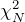) determines the probability of a normal distribution where the mean value (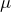) equals 0 and variance (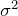) equals 1.

Figure 3.15 provides examples of theprobability density function (PDF) for different values of N. As N approaches infinity, thedistribution converges with the normal distribution. For all thedistributions, the mean value is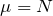and the variance is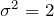.

The formula for the PDF is as follows (Equation 16), where Γ is the Gamma function.

(1)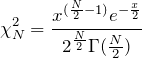Equation 16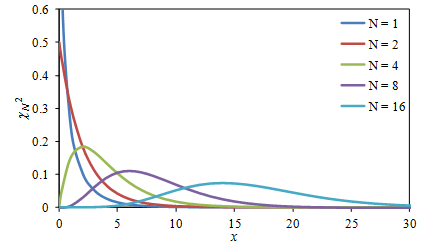Figure 3.14.distribution for the sum of N values of x2.

The squared values used to evaluate the mean-square are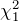distributed. This is illustrated in Figure 3.15 using the car vibration signal shown in Figure 3.2. The distribution is strongly biased toward small values as shown by the simplified formula for:

(2)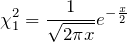Equation 17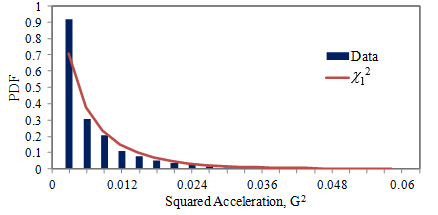Figure 3.15. PDF of squared car vibration data from Figure 3.2 compared to.

As variance is derived from the mean-square value, the confidence interval for the variance can be determined using thedistribution.

For a random variable x with a standard deviation of σx, the summation of N values-squared has a σx2χ12 distribution. Therefore, the variance has a σx2χ12/N distribution.

As thedistributions are not symmetrical, the estimated confidence intervals for the variance are not symmetrical. In Figure 3.16, the values of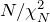are plotted versus N for different confidence levels. The confidence interval for the variance is then stated as the interval:

(3)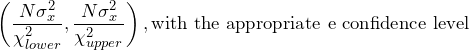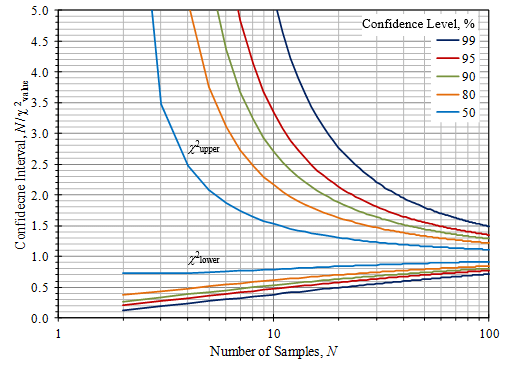Figure 3.16. Confidence intervals using thedistribution for small sample sizes.

For N > 100, normal distribution can be used. The standard deviation of the variance estimate is √2/N σxand the (symmetrical) confidence intervals from Figure 3.13 can be used.